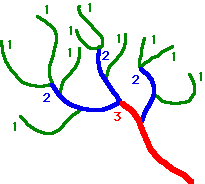PhysicalGeography.net | FUNDAMENTALS eBOOK

CHAPTER 10: Introduction to the Lithosphere

# (ab). Stream Morphometry

Morphometry is defined as the measurement of the shape. Morphometric studies in the field of hydrology were first initiated by R.E. Horton and A.E. Strahler in the 1940s and 1950s. The main purpose of this work was to discover holistic stream properties from the measurement of various stream attributes.

One of the first attributes to be quantified was the hierarchy of stream segments according to an ordering classification system (Figure 10ab-1). In this system, channel segments were ordered numerically from a stream's headwaters to a point somewhere down stream. Numerical ordering begins with the tributaries at the stream's headwaters being assigned the value 1. A stream segment that resulted from the joining of two 1st order segments was given an order of 2. Two 2nd order streams formed a 3rd order stream, and so on. Analysis of this data revealed some interesting relationships. For example, the ratio between the number of stream segments in one order and the next, called the bifurcation ratio, was consistently around three. R.E. Horton called this association the law of stream numbers.Order

Number of Segments

Bifurcation Ratio

1

10

 3.33 3

2

3

3

1

Figure 10ab-1: Example of stream ordering and the calculation of bifurcation ratio.

R.E. Horton applied morphometric analysis to a variety of stream attributes and from these studies he proposed a number of laws of drainage composition. Horton's law of stream lengths suggested that a geometric relationship existed between the number of stream segments in successive stream orders. The law of basin areas indicated that the mean basin area of successive ordered streams formed a linear relationship when graphed. The results described above and the outcomes of other related analyses convinced researchers that these findings suggested that some underlying factor (or factors) was governing the structure of the various stream attributes in a similar predictable way. Studies of other natural branching networks have revealed patterns similar to the stream order model. For example, the bifurcation ratio of three has also been discovered in the rooting systems of plants, the branching structure of woody plants, and the veination in leaves and the human circulatory system.

In addition to the mathematical relationships found in stream ordering, various aspects of drainage network forms were also found to be quantifiable. One such relationship was drainage density. Drainage density is a measure of the length of stream channel per unit area of drainage basin. Mathematically it is expressed as:

Drainage Density (Dd) = Stream Length / Basin Area

The measurement of drainage density provides a hydrologist or geomorphologist with a useful numerical measure of landscape dissection and runoff potential. On a highly permeable landscape, with small potential for runoff, drainage densities are sometimes less than 1 kilometer per square kilometer. On highly dissected surfaces densities of over 500 kilometers per square kilometer are often reported. Closer investigations of the processes responsible for drainage density variation have discovered that a number of factors collectively influence stream density. These factors include climate, topography, soil infiltration capacity, vegetation, and geology.

Study Guide